# Padovan and Fibonacci Spirals

Start with an equilateral triangle with side length 1, and place a second unit triangle next to it. At each stage, moving counterclockwise around the resulting figure, construct an equilateral triangle whose base falls along the current side.

Here are the first three triangles, all with side length 1.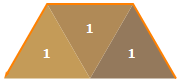Next, place an equilateral triangle with side length 2 on the bottom of the figure.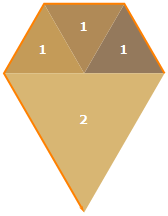The next triangle also has side length 2, and the one after that has side length 3. The orange arrow following the outer edge of the figure is starting to look like a spiral.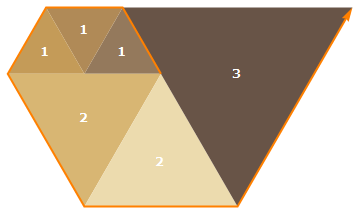Here’s what it looks like after 12 triangles.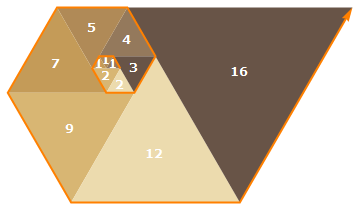Continuing the process, the triangle side lengths 1, 1, 1, 2, 2, 3, 4, 5, 7, 9, 12, 16, 21, …, form a sequence called the Padovan spiral numbers, which are normally defined with the relation P(n) = P(n−2) + P(n−3), with initial conditions P(0) = P(1) = P(2) = 1. (This is OEIS A134816.)

Starting instead with a unit square, place another unit square next to it. At each stage, moving counterclockwise around the figure, construct a square with side length matching the current side of the resulting figure. The square side lengths match the Fibonacci numbers, traditionally defined with the relation Fn = Fn−1 + Fn−2, with initial conditions F1 = F2 = 1.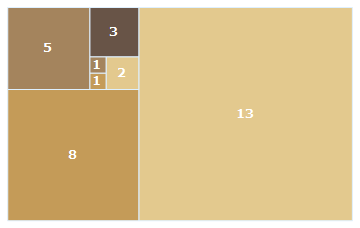The figure also arises in continued fraction expansions of ratios of successive Fibonacci numbers.

A similar process can be carried out in three dimensions, beginning with two 1×1×1 cubes: continue around the figure, placing cuboids to the left, back, top, right, front, and bottom (in that order) of each step of the resulting figure. At each stage, the large cuboid side lengths form the Padovan spiral number sequence we saw above with triangles.